Courses

# Test: Arithmetic - 5 (2013-2010)

## 20 Questions MCQ Test UPSC Topic Wise Previous Year Questions | Test: Arithmetic - 5 (2013-2010)

Description
This mock test of Test: Arithmetic - 5 (2013-2010) for UPSC helps you for every UPSC entrance exam. This contains 20 Multiple Choice Questions for UPSC Test: Arithmetic - 5 (2013-2010) (mcq) to study with solutions a complete question bank. The solved questions answers in this Test: Arithmetic - 5 (2013-2010) quiz give you a good mix of easy questions and tough questions. UPSC students definitely take this Test: Arithmetic - 5 (2013-2010) exercise for a better result in the exam. You can find other Test: Arithmetic - 5 (2013-2010) extra questions, long questions & short questions for UPSC on EduRev as well by searching above.
QUESTION: 1

### There are some balls of red, green and yellow colour lying on a table. There are as many red balls as there are yellow balls. There are twice as many yellow balls as there are green ones. The number of red balls 

Solution:

According to question
R = y and y = 2G
∴ R = 2G
Hence, the number of red balls is double the number of green balls.

QUESTION: 2

### The tank-full pertrol in Arun’s motor-cycle lasts for 10 days. If he starts using 25% more everyday, how many days will the tank-full pertrol last? 

Solution:

Let ‘x’ be the initial petrol consumption. Let ‘y’ be the number of days petrol lasts for. According to question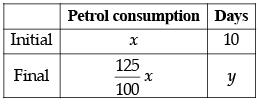Hence, it’s a case of indirect variation.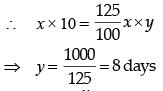QUESTION: 3

### In a garrison, there was food for 1000 soldiers for one month. After 10 days, 1000 more soldiers joined the garrison. How long would the soldiers be able to carry on with the remaining food? 

Solution:

Let the remaining food last for x days.
1000 soldiers had provisions for (30 – 10) = 20 days
(1000 + 1000) men had provisions for x days.
More men, less days (indirect proportion)
∴ 2000 : 1000 : : 20 : x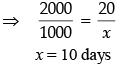QUESTION: 4

Out of 120 applications for a post, 70 are male and 80 have a driver’s license. What is the ratio between the minimum to maximum number of males having driver’s license?



Solution:

Out of 120, there are 70 males.
∴ 120 – 70 = 50 females
For a maximum, all 70 male shall have driver’s license.
For a minimum, if all 50 females posses driver’s license, then the remaining 30 licenses, shall be possessed by males.
So minimum male driver’s licenses = 30.
Min. to max. = 30 to 70 = 3 to 7

QUESTION: 5

A sum of ₹ 700 has to be used to give seven cash prizes to the students of a school for their overall academic performance. If each prize is ₹ 20 less than its preceding prize, what is the least value of the prize?



Solution:

Let the least value be x. Then the next value is x + 20 and the next value is x + 40 and so on.
According to question
(x) + (x + 20) + (x + 40) + (x + 60) + (x + 80) + (x + 100) + (x + 120) = 700
⇒ 7x + (20 + 40 + 60 + 80 + 100 + 120) = 700
⇒ 7x + 20 (1 + 2 + 3 + 4 + 5 + 6) = 700
⇒ 7x + 20 × 21 = 700
⇒ 7(x + 60) = 7(100)
⇒ x = 40

QUESTION: 6

A gardener has 1000 plants. He wants to plant them in such a way that the number of rows and the number of columns remains the same. What is the minimum number of plants that he needs more for this purpose?



Solution:

If the number of rows and columns are to be equal, then the total number of trees would represent a perfect square. Since, 1000 is not a perfect square, we need to check for a perfect square above and nearest to 1000.
It’s 1024, which is square of 32. So he needs 24 more trees to get 1024.

QUESTION: 7

In a rare coin collection, there is one gold coin for every three non-gold coins. 10 more gold coins are added to the collection and the ratio of gold coins to non-gold coins would be 1 : 2. Based on the information, the total number of coins in the collection now becomes



Solution:

Let gold coin be represented by ‘G’.
Let non-gold coin be represented by ‘N’.
Initial ratio = G/N = 1/3  ...(i)
After adding 10 gold coins, the new ratio will be (G+10)/N = 1/2  ...(ii)
Putting the value of G = N/3 in equation (2).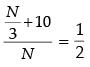On solving, we get N = 60
and G = 30
Presently, the total number of coins in the collection = 10 + 20 + 60 = 90

QUESTION: 8

In a group of persons, 70% of the persons are male and 30% of the persons are married. If two-sevenths of the males are married, what fraction of the females is single?



Solution:

Let the no. of total persons = 100
No. of male = 70%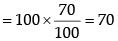∴ No. of female = 100 – 70 = 30
Total married person = 30 %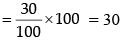∴ Total unmarried person = 100–30 =70
Married male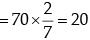∴ Married female = 30 – 20 = 10
Now, unmarried female = 30 – 10 = 20
∴ Required fraction = 20/30 = 2/3

QUESTION: 9

A student on her first 3 tests received an average score of N points. If she exceeds her previous average score by 20 points on her fourth test, then what is the average score for the first 4 tests?



Solution:

Average score for the first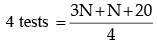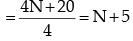QUESTION: 10

Three persons start walking together and their steps measure 40 cm, 42 cm and 45 cm respectively. What is the minimum distance each should walk so that each can cover the same distance in complete steps?



Solution:

Required minimum distance = L. C. M of 40, 42 and 45.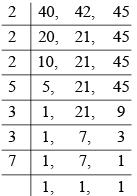L.C.M = 2 x 2 x 2 x 5 x 3 x 3 x 7 = 2520
Required distance = 2520÷100 = 25 m  20 cm

QUESTION: 11

A person has only ₹ 1 and ₹ 2 coins with her. If the total number of coins that she has is 50 and the amount of money with her is ₹ 75, then the number of ₹ 1 and ₹ 2 coins are, respectively



Solution:

Let the no. of ₹ 1 coins = x
and the no. of ₹ 2 coins = y
According to the question:
x + y = 50 ... (i)
x.1 + 2. y = 75 ... (ii)
on solving (i) and (ii), we get
2y – y = 75 – 50
⇒ y = 25
put value of y in equation (i), we get
x = 50 – y
= 50 – 25 = 25
Hence, number of  ₹ 1 and  ₹ 2 coins are 25 and 25 respectively.

QUESTION: 12

Consider the following figure and answer the item that follows: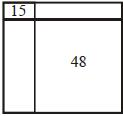A square is divided into four rectangles as shown above. The lengths of the sides of rectangles are natural numbers. The areas of two rectangles are indicated in the figure. What is the length of each side of the square?



Solution: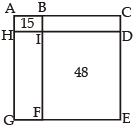Area of ABIH = 15 ⇒ AH = 3 and AB = 5
Now, area of EFID = 48 ⇒ possibilities of length and breadth are (1,48), (2,24), (3,16), (4,12), (6,8)
Since, BC > AB ⇒ BC > 5
Now, BC = ID ⇒ BC = ID = 6.
Hence, length of square = 11

QUESTION: 13

A contract on construction job specifies a penalty for delay in completion of the work beyond a certain date is as follows: ₹ 200 for the first day, ₹ 250 for the second day, ₹ 300 for the third day etc., the penalty for each succeeding day being ₹ 50 more than that of the preceding day. How much penalty should the contractor pay if he delays the work by 10 days?



Solution:

Given series is 200,250,300, .....n
Here, a = first term = 200
d = common difference = 50
n = 10
Since, given series is A.P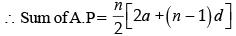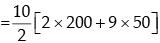= 5 x 850 = 4250
Required penalty = sum of the series = ₹ 4250.

QUESTION: 14

A candidate attempted 12 questions and secured full marks in all of them. If he obtained 60% in the test and all questions carried equal marks, then what is the number of questions in the test?



Solution:

Let total no. of questions be x.
Now, Right questions = 12 = 60% of x.
0.6x = 12
x = 20

QUESTION: 15

The difference between the simple interest received from two banks on ₹500 for two years is ₹ 2.50. What is the difference between their rates?



Solution:

Let the two interest rates be R1 and R2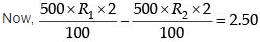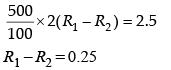QUESTION: 16

A man fills a basket will eggs in such a way that the number of eggs added on each successive day is the same as the number already present in the basket. This way the basket gets completely filled in 24 day. After how many days the basket was (1/4)th full?



Solution:

Let x be the no. of eggs already present in the basket.
Then, no. of eggs on each successive days:
x, 2x, 4x, 8x, ............ upto 24 days.
From here, we can see that number of eggs are getting doubled on each successive days.
On 24th day basket was filled completely.
∴ On 23rd day basket was filled = 1/2
Hence, on 22nd day basket was filled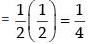QUESTION: 17

Each person’s performance compared with all other persons is to be done to rank them subjectively. How many comparisons are needed in total, if there are 11 persons?



Solution:

lst person is to be compared with the other 10 persons i.e 10 comparison. 2nd person is to be compared with the remaining 9 persons and so on.
Hence, total no. of comparison
= 10 + 9 + ...... + 1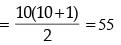QUESTION: 18

Half of the villagers of a certain village have their own houses. One-fifth of the villagers cultivate paddy. One-third of the villagers are literate. Four-fifth of the villagers are below twenty five. Then, which one of the following is certainly true?



Solution:

Own house paddyliterate (< 25)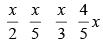where, x = total no. of villagers.
All the villagers who have their own houses are 50, while no. of literate villagers are 33, so (a) is not true.
No. of villagers under 25 is 80, while literate are 33, so clearly some of them under 25 are literate.
Thus, option (b) is correct.
Similarly (c) and (d) also, are not true.

QUESTION: 19

In a group of five persons A, B, C, D and E, there is a professor, a doctor and lawyer. A and D are unmarried ladies, and do not work. Of the married couple in the Group, E is the husband. B is the brother of A and is neither a doctor nor a lawyer. Who is the professor?



Solution:

As, A and D do not work, so any of B, C or E could be the professor, doctor or lawyer. Now, B is neither a doctor or lawyer, so clearly B is the professor.

QUESTION: 20

How many numbers from 0 to 999 are not divisible by either 5 or 7?



Solution:

Numbers from (0 – 999) divisible by 7,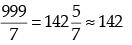Numbers from (0 – 999) divisible by 5,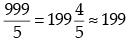There are few numbers which are divisible by both 5 and 7, i.e., by 35.
Numbers from (0 – 999) divisible by 35,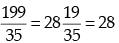Numbers divisible by 5 or 7 = 142 + 199 – 28 = 313
Hence, total numbers between (0 – 999) not divisible by 5 or 7 = 999 – 313 = 686